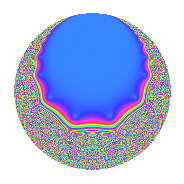Properties

 Label 8.14.a.bLevel 8 Weight 14 Character orbit 8.a Self dual Yes Analytic conductor 8.578 Analytic rank 0 Dimension 2 CM No Inner twists 1

Related objects

Newspace parameters

 Level: $$N$$ = $$8 = 2^{3}$$ Weight: $$k$$ = $$14$$ Character orbit: $$[\chi]$$ = 8.a (trivial)

Newform invariants

 Self dual: Yes Analytic conductor: $$8.57847431615$$ Analytic rank: $$0$$ Dimension: $$2$$ Coefficient field: $$\Q(\sqrt{781})$$ Coefficient ring: $$\Z[a_1, a_2, a_3]$$ Coefficient ring index: $$2^{7}$$ Fricke sign: $$-1$$ Sato-Tate group: $\mathrm{SU}(2)$

$q$-expansion

Coefficients of the $$q$$-expansion are expressed in terms of $$\beta = 64\sqrt{781}$$. We also show the integral $$q$$-expansion of the trace form.

 $$f(q)$$ $$=$$ $$q$$ $$+ ( 436 - \beta ) q^{3}$$ $$+ ( 9238 - 12 \beta ) q^{5}$$ $$+ ( 55464 + 222 \beta ) q^{7}$$ $$+ ( 1794749 - 872 \beta ) q^{9}$$ $$+O(q^{10})$$ $$q$$ $$+ ( 436 - \beta ) q^{3}$$ $$+ ( 9238 - 12 \beta ) q^{5}$$ $$+ ( 55464 + 222 \beta ) q^{7}$$ $$+ ( 1794749 - 872 \beta ) q^{9}$$ $$+ ( 8237020 - 531 \beta ) q^{11}$$ $$+ ( 9372286 + 10452 \beta ) q^{13}$$ $$+ ( 42415480 - 14470 \beta ) q^{15}$$ $$+ ( -76896814 - 38184 \beta ) q^{17}$$ $$+ ( -59373820 + 93819 \beta ) q^{19}$$ $$+ ( -685990368 + 41328 \beta ) q^{21}$$ $$+ ( 359134456 - 111462 \beta ) q^{23}$$ $$+ ( -674709937 - 221712 \beta ) q^{25}$$ $$+ ( 2876892808 - 580618 \beta ) q^{27}$$ $$+ ( 154670670 + 2374788 \beta ) q^{29}$$ $$+ ( 2883752096 + 1482936 \beta ) q^{31}$$ $$+ ( 5289996976 - 8468536 \beta ) q^{33}$$ $$+ ( -8009695632 + 1385268 \beta ) q^{35}$$ $$+ ( -5810776650 + 10246116 \beta ) q^{37}$$ $$+ ( -29349380456 - 4815214 \beta ) q^{39}$$ $$+ ( 655584138 + 8079216 \beta ) q^{41}$$ $$+ ( -14797810052 - 9339771 \beta ) q^{43}$$ $$+ ( 50053976126 - 29592524 \beta ) q^{45}$$ $$+ ( 6156808944 + 16143540 \beta ) q^{47}$$ $$+ ( 63845578073 + 24626016 \beta ) q^{49}$$ $$+ ( 88622688680 + 60248590 \beta ) q^{51}$$ $$+ ( -19003003514 - 50827740 \beta ) q^{53}$$ $$+ ( 96477465832 - 103749618 \beta ) q^{55}$$ $$+ ( -326011714864 + 100278904 \beta ) q^{57}$$ $$+ ( 126672955852 - 136967391 \beta ) q^{59}$$ $$+ ( -323622192146 + 14150004 \beta ) q^{61}$$ $$+ ( -519726611448 + 350069670 \beta ) q^{63}$$ $$+ ( -314647187756 - 15911856 \beta ) q^{65}$$ $$+ ( 809996903156 - 36810993 \beta ) q^{67}$$ $$+ ( 513146885728 - 407731888 \beta ) q^{69}$$ $$+ ( -520135071256 - 352709874 \beta ) q^{71}$$ $$+ ( 2002641954346 - 86109192 \beta ) q^{73}$$ $$+ ( 415077834380 + 578043505 \beta ) q^{75}$$ $$+ ( 79756388448 + 1799167056 \beta ) q^{77}$$ $$+ ( -1260888786032 - 525520788 \beta ) q^{79}$$ $$+ ( 250298701529 - 1739792600 \beta ) q^{81}$$ $$+ ( -145243115452 - 996493749 \beta ) q^{83}$$ $$+ ( 755423627276 + 570017976 \beta ) q^{85}$$ $$+ ( -7529453404968 + 880736898 \beta ) q^{87}$$ $$+ ( -4361877828870 - 639382536 \beta ) q^{89}$$ $$+ ( 7942549238448 + 2660357220 \beta ) q^{91}$$ $$+ ( -3486560759680 - 2237192000 \beta ) q^{93}$$ $$+ ( -4149992101288 + 1579185762 \beta ) q^{95}$$ $$+ ( 4800856149986 + 4881770040 \beta ) q^{97}$$ $$+ ( 16264611663212 - 8135693159 \beta ) q^{99}$$ $$+O(q^{100})$$ $$\operatorname{Tr}(f)(q)$$ $$=$$ $$2q$$ $$\mathstrut +\mathstrut 872q^{3}$$ $$\mathstrut +\mathstrut 18476q^{5}$$ $$\mathstrut +\mathstrut 110928q^{7}$$ $$\mathstrut +\mathstrut 3589498q^{9}$$ $$\mathstrut +\mathstrut O(q^{10})$$ $$2q$$ $$\mathstrut +\mathstrut 872q^{3}$$ $$\mathstrut +\mathstrut 18476q^{5}$$ $$\mathstrut +\mathstrut 110928q^{7}$$ $$\mathstrut +\mathstrut 3589498q^{9}$$ $$\mathstrut +\mathstrut 16474040q^{11}$$ $$\mathstrut +\mathstrut 18744572q^{13}$$ $$\mathstrut +\mathstrut 84830960q^{15}$$ $$\mathstrut -\mathstrut 153793628q^{17}$$ $$\mathstrut -\mathstrut 118747640q^{19}$$ $$\mathstrut -\mathstrut 1371980736q^{21}$$ $$\mathstrut +\mathstrut 718268912q^{23}$$ $$\mathstrut -\mathstrut 1349419874q^{25}$$ $$\mathstrut +\mathstrut 5753785616q^{27}$$ $$\mathstrut +\mathstrut 309341340q^{29}$$ $$\mathstrut +\mathstrut 5767504192q^{31}$$ $$\mathstrut +\mathstrut 10579993952q^{33}$$ $$\mathstrut -\mathstrut 16019391264q^{35}$$ $$\mathstrut -\mathstrut 11621553300q^{37}$$ $$\mathstrut -\mathstrut 58698760912q^{39}$$ $$\mathstrut +\mathstrut 1311168276q^{41}$$ $$\mathstrut -\mathstrut 29595620104q^{43}$$ $$\mathstrut +\mathstrut 100107952252q^{45}$$ $$\mathstrut +\mathstrut 12313617888q^{47}$$ $$\mathstrut +\mathstrut 127691156146q^{49}$$ $$\mathstrut +\mathstrut 177245377360q^{51}$$ $$\mathstrut -\mathstrut 38006007028q^{53}$$ $$\mathstrut +\mathstrut 192954931664q^{55}$$ $$\mathstrut -\mathstrut 652023429728q^{57}$$ $$\mathstrut +\mathstrut 253345911704q^{59}$$ $$\mathstrut -\mathstrut 647244384292q^{61}$$ $$\mathstrut -\mathstrut 1039453222896q^{63}$$ $$\mathstrut -\mathstrut 629294375512q^{65}$$ $$\mathstrut +\mathstrut 1619993806312q^{67}$$ $$\mathstrut +\mathstrut 1026293771456q^{69}$$ $$\mathstrut -\mathstrut 1040270142512q^{71}$$ $$\mathstrut +\mathstrut 4005283908692q^{73}$$ $$\mathstrut +\mathstrut 830155668760q^{75}$$ $$\mathstrut +\mathstrut 159512776896q^{77}$$ $$\mathstrut -\mathstrut 2521777572064q^{79}$$ $$\mathstrut +\mathstrut 500597403058q^{81}$$ $$\mathstrut -\mathstrut 290486230904q^{83}$$ $$\mathstrut +\mathstrut 1510847254552q^{85}$$ $$\mathstrut -\mathstrut 15058906809936q^{87}$$ $$\mathstrut -\mathstrut 8723755657740q^{89}$$ $$\mathstrut +\mathstrut 15885098476896q^{91}$$ $$\mathstrut -\mathstrut 6973121519360q^{93}$$ $$\mathstrut -\mathstrut 8299984202576q^{95}$$ $$\mathstrut +\mathstrut 9601712299972q^{97}$$ $$\mathstrut +\mathstrut 32529223326424q^{99}$$ $$\mathstrut +\mathstrut O(q^{100})$$

Embeddings

For each embedding $$\iota_m$$ of the coefficient field, the values $$\iota_m(a_n)$$ are shown below.

For more information on an embedded modular form you can click on its label.

Label $$\iota_m(\nu)$$ $$a_{2}$$ $$a_{3}$$ $$a_{4}$$ $$a_{5}$$ $$a_{6}$$ $$a_{7}$$ $$a_{8}$$ $$a_{9}$$ $$a_{10}$$
1.1
 14.4732 −13.4732
0 −1352.57 0 −12224.8 0 452526. 0 235118. 0
1.2 0 2224.57 0 30700.8 0 −341598. 0 3.35438e6 0
 $$n$$: e.g. 2-40 or 990-1000 Significant digits: Format: Complex embeddings Normalized embeddings Satake parameters Satake angles

Inner twists

This newform does not admit any (nontrivial) inner twists.

Atkin-Lehner signs

$$p$$ Sign
$$2$$ $$-1$$

Hecke kernels

This newform can be constructed as the kernel of the linear operator $$T_{3}^{2}$$ $$\mathstrut -\mathstrut 872 T_{3}$$ $$\mathstrut -\mathstrut 3008880$$ acting on $$S_{14}^{\mathrm{new}}(\Gamma_0(8))$$.